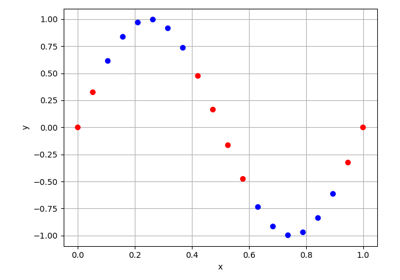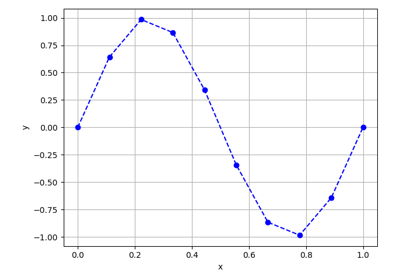gemseo / datasets

# dataset module¶

A generic data structure with entries and variables.

The concept of dataset is a key element for machine learning, post-processing, data analysis, …

A Dataset is a pandas MultiIndex DataFrame storing series of data representing the values of multidimensional features belonging to different groups of features.

A Dataset can be set either from a file (from_csv() and from_txt()) or from a NumPy array (from_array()), and can be enriched from a group of variables (add_group()) or from a single variable (add_variable()).

An AbstractFullCache or an OptimizationProblem can also be exported to a Dataset using the methods AbstractFullCache.to_dataset() and OptimizationProblem.to_dataset().

class gemseo.datasets.dataset.Dataset(data=None, index=None, columns=None, dtype=None, copy=None, *, dataset_name='')[source]

Bases: DataFrame

A generic data structure with entries and variables.

A variable is defined by a name and a number of components. For instance, the variable "x" can have 2 components: 0 and 1. Or the variable y can have 4 components: "a", "b", "c" and "d".

A variable belongs to a group of variables (default: DEFAULT_GROUP). Two variables can have the same name; only the tuple (group_name, variable_name) is unique and is therefore called a variable identifier.

Based on a set of variable identifiers, Dataset is a collection of entries corresponding to a set of variable identifiers. An entry corresponds to an index of the Dataset.

A Dataset is a special pandas DataFrame with the multi-index (group_name, variable_name, component). It must be built from the methods add_variable(), add_group(), from_array(), from_txt() and from_csv().

Miscellaneous information that is not specific to an entry of the dataset can be stored in the dictionary misc, as dataset.misc["year"] = 2023.

Warning

A Dataset behaves like any multi-index DataFrame but its instantiation using the constructor dataset = Dataset(data, ...) can lead to some inconsistencies (multi-index levels, index values, dtypes, …). Hence, the construction from the dedicated methods is recommended, e.g. dataset = Dataset(); dataset.add_variable("x", data).

Notes

The columns of a data structure (NumPy array, DataFrame, Dataset, …) are called features. The features of a Dataset include all the components of all the variables of all the groups.

Initialize self. See help(type(self)) for accurate signature.

Parameters:
• dataset_name (str) –

The name of the dataset.

By default it is set to “”.

• data (ndarray | Iterable | dict | DataFrame | None) –

• index (Axes | None) –

• columns (Axes | None) –

• dtype (Dtype | None) –

• copy (bool | None) –

Add the data related to a new group.

Parameters:
• group_name (str) – The name of the group.

• data (DataType) – The data.

• variable_names (StrColumnType) –

The names of the variables. If empty, use DEFAULT_VARIABLE_NAME.

By default it is set to ().

• variable_names_to_n_components (dict[str, int] | None) – The number of components of the variables. If variable_names is empty, this argument is not considered. If None, assume that all the variables have a single component.

Raises:

ValueError – If the group already exists.

Return type:

None

Add the data related to a variable.

Parameters:
• variable_name (str) – The name of the variable.

• data (ndarray | Iterable[Any] | Any) – The data.

• group_name (str) –

The name of the group related to this variable.

By default it is set to “parameters”.

• components (int | Iterable[int]) –

The component(s) considered. If empty, use [0, ..., n_features].

By default it is set to ().

Return type:

None

Warning

The shape of data must be consistent with the number of entries of the dataset. If the dataset is empty, the number of entries will be deducted from data.

Notes

The data can be passed as:

• an array shaped as (n_entries, n_features),

• an array shaped as (1, n_features) that will be reshaped as (n_entries, n_features) by replicating the original array n_entries times,

• an array shaped as (n_entries,) that will be reshaped as (n_entries, 1),

• a scalar that will be converted into an array shaped as (n_entries, 1) if components is empty or (n_entries, n_features) where n_features will be deducted from components.

Raises:

ValueError – If the group already has the added components of the variable named variable_name.

Parameters:
Return type:

None

classmethod from_array(data, variable_names=(), variable_names_to_n_components=None, variable_names_to_group_names=None)[source]

Create a dataset from a NumPy array.

Parameters:
• data (DataType) – The data to be stored, with the shape (n_entries, n_components).

• variable_names (StrColumnType) –

The names of the variables. If empty, use default names.

By default it is set to ().

• variable_names_to_n_components (dict[str, int] | None) – The number of components of the variables. If None, assume that all the variables have a single component.

• variable_names_to_group_names (dict[str, str] | None) – The groups of the variables. If None, use Dataset.DEFAULT_GROUP for all the variables.

Returns:

A dataset built from the NumPy array.

Return type:

Dataset

classmethod from_csv(file_path, delimiter=',')[source]

Set the dataset from a CSV file.

The first three rows contain the values of the multi-index (column_name, variable_name, component).

If the file does not contain multi-index information and not just an array, the method from_txt() is better suited.

Parameters:
• file_path (Path | str) – The path to the file containing the data.

• delimiter (str) –

The field delimiter.

By default it is set to “,”.

Returns:

A dataset built from the CSV file.

Return type:

Dataset

classmethod from_txt(file_path, variable_names=(), variable_names_to_n_components=None, variable_names_to_group_names=None, delimiter=',', header=True)[source]

Create a dataset from a text file.

If the file contains multi-index information and not just an array, the from_csv() method is better suited.

Parameters:
• file_path (Path | str) – The path to the file containing the data.

• variable_names (Iterable[str]) –

The names of the variables. If empty and header is True, read the names from the first line of the file. If empty and header is False, use default names based on the patterns the DEFAULT_NAMES associated with the different groups.

By default it is set to ().

• variable_names_to_n_components (dict[str, int] | None) – The number of components of the variables. If None, assume that all the variables have a single component.

• variable_names_to_group_names (dict[str, str] | None) – The groups of the variables. If None, use DEFAULT_GROUP for all the variables.

• delimiter (str) –

The field delimiter.

By default it is set to “,”.

Whether to read the names of the variables on the first line of the file.

By default it is set to True.

Returns:

A dataset built from the text file.

Return type:

Dataset

get_columns(variable_names=(), as_tuple=False)[source]

Return the columns based on variable names.

Parameters:
• variable_names (Iterable[str]) –

The names of the variables. If empty, use all the variables.

By default it is set to ().

• as_tuple (bool) –

Whether the variable identifiers are returned as tuples.

By default it is set to False.

Returns:

The columns, either as a variable identifier (group_name, variable_name, component) or as a variable component name "variable_name[component]" (or "variable_name" if the dimension of the variable is 1).

Return type:

list[str]

get_group_names(variable_name)[source]

Return the names of the groups that contain a variable.

Parameters:

variable_name (str) – The name of the variable.

Returns:

The names of the groups that contain the variable.

Return type:

list[str]

get_normalized(excluded_variable_names=(), excluded_group_names=(), use_min_max=True, center=False, scale=False)[source]

Return a normalized copy of the dataset.

Parameters:
• excluded_variable_names (str | Iterable[str]) –

The names of the variables not to be normalized. If empty, normalize all the variables.

By default it is set to ().

• excluded_group_names (str | Iterable[str]) –

The names of the groups not to be normalized. If empty, normalize all the groups.

By default it is set to ().

• use_min_max (bool) –

Whether to use the geometric normalization $$(x-\min(x))/(\max(x)-\min(x))$$.

By default it is set to True.

• center (bool) –

Whether to center the variables so that they have a zero mean.

By default it is set to False.

• scale (bool) –

Whether to scale the variables so that they have a unit variance.

By default it is set to False.

Returns:

A normalized dataset.

Return type:

Dataset

get_variable_components(group_name, variable_name)[source]

Return the components of a given variable.

Parameters:
• group_name (str) – The name of the group.

• variable_name (str) – The name of the variable.

Return type:

list[int]

Notes

Assure compatibility pandas 1 and 2 by returning an empty list if KeyError is raised.

Returns:

The components of the variables.

Parameters:
• group_name (str) –

• variable_name (str) –

Return type:

list[int]

get_variable_names(group_name)[source]

Return the names of the variables contained in a group.

Parameters:

group_name (str) – The name of the group.

Return type:

list[str]

Notes

Assure compatibility pandas 1 and 2 by returning an empty list if KeyError is raised.

Returns:

The names of the variables contained in the group.

Parameters:

group_name (str) –

Return type:

list[str]

get_view(group_names=(), variable_names=(), components=(), indices=())[source]

Return a specific group of rows and columns of the dataset.

Parameters:
• group_names (str | Iterable[str]) –

The name(s) of the group(s). If empty, consider all the groups.

By default it is set to ().

• variable_names (str | Iterable[str]) –

The name(s) of the variables(s). If empty, consider all the variables of the considered groups.

By default it is set to ().

• components (int | Iterable[int]) –

The component(s). If empty, consider all the components of the considered variables.

By default it is set to ().

• indices (str | int | Iterable[str | int]) –

The index (indices) of the dataset into which these data is to be inserted. If empty, consider all the indices.

By default it is set to ().

Return type:

Dataset

Notes

The order asked by the user is preserved for the returned Dataset. See also loc().

Returns:

The specific group of rows and columns of the dataset.

Parameters:
Return type:

Dataset

rename_group(group_name, new_group_name)[source]

Rename a group.

Parameters:
• group_name (str) – The group to rename.

• new_group_name (str) – The new group name.

Return type:

None

rename_variable(variable_name, new_variable_name, group_name='')[source]

Rename a variable.

Parameters:
• variable_name (str) – The name of the variable.

• new_variable_name (str) – The new name of the variable.

• group_name (str) –

The group of the variable. If empty, change the name of all the variables matching variable_name.

By default it is set to “”.

Return type:

None

to_dict_of_arrays(by_group=True)[source]

Convert the dataset into a dictionary of NumPy arrays.

Parameters:

by_group (bool) –

Whether the data are returned as {group_name: {variable_name: variable_value}}. Otherwise, the data are returned either as {variable_name: variable_value} if only one group contains the variable variable_name or as {f"{group_name}:{variable_name}": variable_value} if at least two groups contain the variable variable_name.

By default it is set to True.

Returns:

The dataset expressed as a dictionary of NumPy arrays.

Return type:

dict[str, ndarray | dict[str, ndarray]]

transform_data(transformation, group_names=(), variable_names=(), components=(), indices=())[source]

Transform some data of the dataset.

Parameters:
• transformation (Callable[[ndarray], ndarray]) – The function transforming the variable, e.g. "lambda x: 2*x".

• group_names (str | Iterable[str]) –

The name(s) of the group(s) corresponding to these data. If empty, consider all the groups.

By default it is set to ().

• variable_names (str | Iterable[str]) –

The name(s) of the variables(s) corresponding to these data. If empty, consider all the variables of the considered groups.

By default it is set to ().

• components (int | Iterable[int]) –

The component(s) corresponding to these data. If empty, consider all the components of the considered variables.

By default it is set to ().

• indices (str | int | Iterable[str | int]) –

The index (indices) of the dataset corresponding to these data. If empty, consider all the indices.

By default it is set to ().

Return type:

None

update_data(data, group_names=(), variable_names=(), components=(), indices=())[source]

Replace the data of some existing indices and columns by a deepcopy of data.

Parameters:
• data (ndarray | Iterable[Any] | Any) – The new data to be inserted in the dataset.

• group_names (str | Iterable[str]) –

The name(s) of the group(s) corresponding to these data. If empty, consider all the groups.

By default it is set to ().

• variable_names (str | Iterable[str]) –

The name(s) of the variables(s) corresponding to these data. If empty, consider all the variables of the considered groups.

By default it is set to ().

• components (int | Iterable[int]) –

The component(s) corresponding to these data. If empty, consider all the components of the considered variables.

By default it is set to ().

• indices (str | int | Iterable[str | int]) –

The index (indices) of the dataset into which these data is to be inserted. If empty, consider all the indices.

By default it is set to ().

Return type:

None

Notes

Changing the type of data can turn a view into a copy.

DEFAULT_GROUP: ClassVar[str] = 'parameters'

The default group name for the variables.

DEFAULT_VARIABLE_NAME: ClassVar[str] = 'x'

The default name for the variable set with add_group().

The group name for the gradients.

PARAMETER_GROUP: Final[str] = 'parameters'

The group name for the parameters.

property group_names: list[str]

The names of the groups of variables in alphabetical order.

property group_names_to_n_components: dict[str, int]

The names of the groups bound to their number of components.

misc: dict[str, Any]

Miscellaneous information specific to the dataset, and not to an entry.

name: str

The name of the dataset.

property variable_identifiers: list[tuple[str, str]]

The variable identifiers.

A variable identifier is the tuple (group_name, variable_name).

Notes

A variable name can belong to more than one group while a variable identifier is unique as a group name is unique.

property variable_names: list[str]

The names of the variables in alphabetical order.

property variable_names_to_n_components: dict[str, int]

The names of the variables bound to their number of components.

## Examples using Dataset¶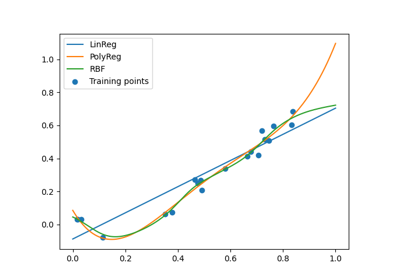Machine learning algorithm selection example

Machine learning algorithm selection example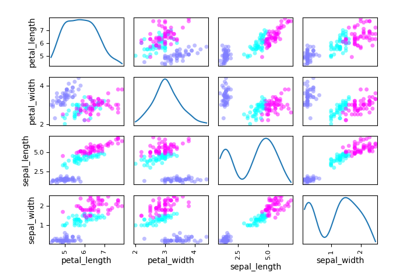Gaussian Mixtures

Gaussian Mixtures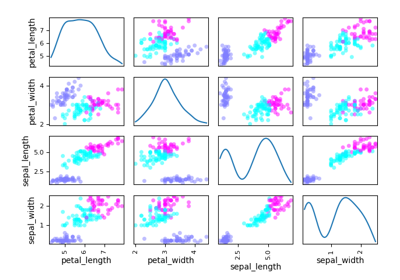K-means

K-meansConvert a cache to a dataset

Convert a cache to a datasetConvert a database to a dataset

Convert a database to a datasetDataset

DatasetDataset from a NumPy array

Dataset from a NumPy arrayThe input-output dataset

The input-output datasetThe optimisation dataset

The optimisation dataset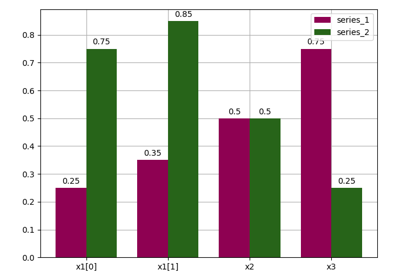Bars

Bars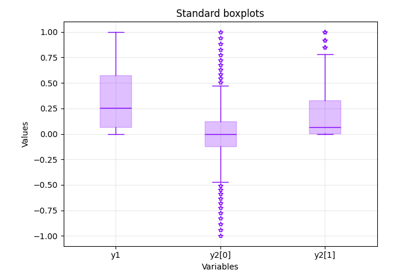Boxplot

Boxplot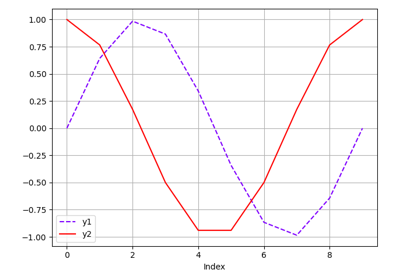Lines

Lines